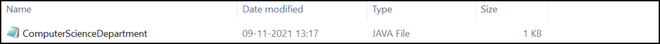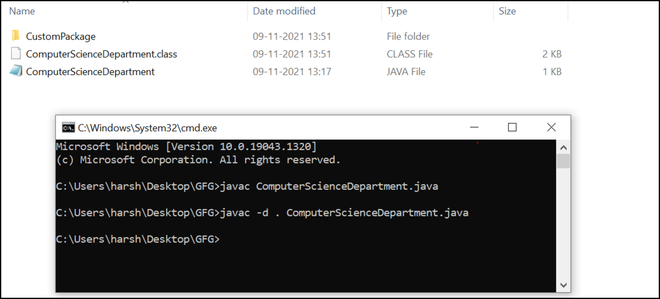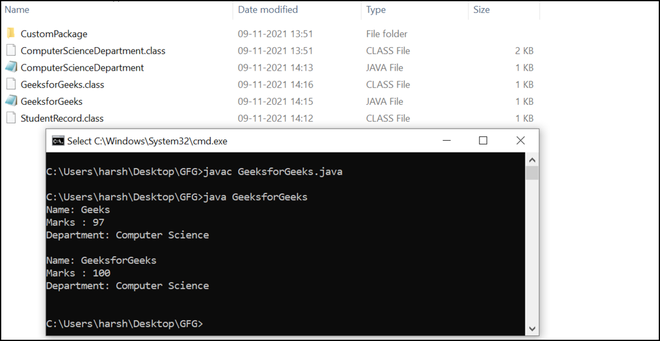# Import Statement in Java

• Last Updated : 13 May, 2022

Import statement in Java is helpful to take a class or all classes visible for a program specified under a package, with the help of a single statement. It is pretty beneficial as the programmer do not require to write the entire class definition. Hence, it improves the readability of the program.

This article focuses on the import statements used in the Java programs and their alternatives.

Syntax 1:

`import package1[.package2].(*);`

Here,

• package1: Top-level package
• package2: Subordinate-level package under package1
• *: To import all the classes

Syntax 2:

`import package1[.package2].(myClass);`

Here,

• package1: Top-level package
• package2: Subordinate-level package under the top-level package
• myClass: Import only myClass

Note: Either we can import a class or we can import all classes specified under the package.

To understand why we do we need to bring a class or classes into visibility, let us consider a Java program without the use of an import statement:

Source Code:

## Java

 `// Java program to demonstrate the ` `// working of a program` `// without any import statement`   `class` `GFG {`   `    ``// Main method` `    ``public` `static` `void` `main(String[] args)` `    ``{` `        ``// Declaring an ArrayList of String type` `        ``ArrayList arrayList` `            ``= ``new` `ArrayList();`   `        ``// Adding elements in the ArrayList` `        ``arrayList.add(``"Geeks"``);` `        ``arrayList.add(``"For"``);` `        ``arrayList.add(``"Geeks"``);`   `        ``// Print the ArrayList` `        ``System.out.println(``"ArrayList: "` `+ arrayList);` `    ``}` `}`

Let us compile the above program:

Compiler Verdict:

```prog.java:11: error: cannot find symbol
ArrayList<String> arrayList
^
symbol:   class ArrayList
location: class GFG

prog.java:12: error: cannot find symbol
= new ArrayList<String>();
^
symbol:   class ArrayList
location: class GFG

2 errors```

Compilation Description: We get the compile-time error. The Javac compiler couldn’t find the ArrayList class in the program. ArrayList class is a part of the java.util package. So we need  to include the ArrayList class defined under Java.util package in our program.

Following are three ways to refer an external class or all external classes specified under a package:

### 1. Fully-qualified name:

The import statement is optional, and we can use the fully-qualified name of the class to refer to a class or package in the program. This method tells the compiler that the class is defined under a particular package, and we want to use that class or classes in our program. Each time we want to use a data member or member function defined in the class, we need to refer to it using a fully-qualified name. It increases the code size of our program and hence makes it less readable. This is the only disadvantage of this method.

Example: Below is the implementation using a fully-qualified name for the class ArrayList defined under Java.util package:

## Java

 `// Java program to demonstrate the working of a program` `// using fully-qualified name or without the use of import` `// statement`   `class` `GFG {`   `    ``// Main method` `    ``public` `static` `void` `main(String[] args)` `    ``{` `        ``// Using fully-qualified name` `        ``// Declaring an ArrayList of String type` `        ``java.util.ArrayList arrayList` `            ``= ``new` `java.util.ArrayList();`   `        ``// Adding elements in the ArrayList` `        ``arrayList.add(``"Geeks"``);` `        ``arrayList.add(``"For"``);` `        ``arrayList.add(``"Geeks"``);`   `        ``// Print the ArrayList` `        ``System.out.println(``"ArrayList: "` `+ arrayList);` `    ``}` `}`

Output

`ArrayList: [Geeks, For, Geeks]`

### 2. Import statement:

An import statement tells the compiler the path of a class or the entire package. It is unlike “#include” in C++, which includes the entire code in the program. Import statement tells the compiler that we want to use a class (or classes) that is defined under a package. It is pretty helpful and recommended over the “fully-qualified name” method as it reduces the overall code size and improves the source code’s readability.

Below is the implementation to illustrate how we can import a class into our program:

## Java

 `// Java program to demonstrate the ` `// working of import statement`   `// Importing ArrayList class specified ` `// under java.util package` `import` `java.util.ArrayList;`   `class` `GFG {`   `    ``// Main method` `    ``public` `static` `void` `main(String[] args)` `    ``{` `        ``// Declaring an ArrayList of String type` `        ``ArrayList arrayList` `            ``= ``new` `ArrayList();`   `        ``// Adding elements in the ArrayList` `        ``arrayList.add(``"Geeks"``);` `        ``arrayList.add(``"For"``);` `        ``arrayList.add(``"Geeks"``);`   `        ``// Print the ArrayList` `        ``System.out.println(``"ArrayList: "` `+ arrayList);` `    ``}` `}`

Output

`ArrayList: [Geeks, For, Geeks]`

Below is the implementation to illustrate how we can import all classes into our program:

Source Code:

## Java

 `// Java program to demonstrate the` `// working of import statement`   `// Importing all classes specified under java.util package` `import` `java.util.*;`   `class` `GFG {`   `    ``// Static function to print array elements` `    ``public` `static` `void` `print(``int` `array[])` `    ``{`   `        ``System.out.print(``"Array: [ "``);`   `        ``for` `(``int` `i = ``0``; i < ``5``; i++)` `            ``System.out.print(array[i] + ``" "``);`   `        ``System.out.print(``"]"``);` `    ``}` `  `  `    ``// main method` `    ``public` `static` `void` `main(String[] args)` `    ``{` `        ``// Declaring an ArrayList of String type` `        ``ArrayList arrayList` `            ``= ``new` `ArrayList();`   `        ``// Adding elements in the ArrayList` `        ``arrayList.add(``"Geeks"``);` `        ``arrayList.add(``"For"``);` `        ``arrayList.add(``"Geeks"``);`   `        ``// Declaring an array of integers` `        ``int` `array[] = { ``10``, ``3``, ``5``, ``11``, ``20` `};`   `        ``// sort function defined under Arrays class` `        ``Arrays.sort(array);`   `        ``// Print the ArrayList` `        ``System.out.println(``"ArrayList: "` `+ arrayList);`   `        ``// Calling print() function` `        ``print(array);` `    ``}` `}`

Output

```ArrayList: [Geeks, For, Geeks]
Array: [ 3 5 10 11 20 ]```

Let us now see the working of the import statement by creating our custom package and a class inside it. We can include the following statement before all class definitions to bundle a program into a package.

#### Syntax:

`package myPackage;`

Here,

• myPackage: Name of the package

Below is the implementation to illustrate how we can import a class by creating our custom package:

## Java

 `// Statement to create a package` `package` `CustomPackage;`   `// Public class` `public` `class` `ComputerScienceDepartment {` `    ``String name;` `    ``int` `marks;`   `    ``// user defined constructor method` `    ``public` `ComputerScienceDepartment(String studentName,` `                                     ``int` `studentMarks)` `    ``{` `        ``// Assigning values to data ` `        ``// members with the help of` `        ``// values passed to the constructor` `        ``name = studentName;` `        ``marks = studentMarks;` `    ``}`   `    ``// Display method to display student's information` `    ``public` `void` `display()` `    ``{` `        ``System.out.println(``"Name: "` `+ name + ``'\n'` `                           ``+ ``"Marks : "` `+ marks + ``'\n'` `                           ``+ ``"Department: "` `                           ``+ ``"Computer Science"` `+ ``'\n'``);` `    ``}` `}`

We have created the above Java source file locally on our system and saved it as ComputerScienceDepartment.javaJava Source Code file

Let us now compile the program using the javac compiler and bundle it as a package through command prompt,

```javac ComputerScienceDepartment.java
javac -d . ComputerScienceDepartment.java```Compilation of the programCustomPackage folder

Compilation Description: As you can see in the above picture, the CustomPackage folder is generated, which contains ComputerScienceDepartment.class byte code file. This file is later executed by a Java interpreter(JVM). Now the package is ready, and we can create another program and import this package into the program.

Below is the source code which includes/imports the ComputerScienceDepartment class defined under CustomPackage in the program:

## Java

 `// Import the public class defined under ` `// the created package (CustomPackage)` `import` `CustomPackage.ComputerScienceDepartment;`   `class` `GeeksforGeeks {`   `    ``// main method` `    ``public` `static` `void` `main(String args[])` `    ``{` `        ``// Initializing a variable by passing name` `        ``// and marks of the student as an argument to` `        ``// the constructor` `        ``ComputerScienceDepartment student1` `            ``= ``new` `ComputerScienceDepartment(``"Geeks"``, ``97``);`   `        ``// Using display() method defined under` `        ``// ComputerScienceDepartment class to print sudent1` `        ``// information` `        ``student1` `            ``.display(); ``// you may also call the display()`   `        ``// Initializing another variable by passing name` `        ``// and marks of the student as an argument to` `        ``// the constructor` `        ``ComputerScienceDepartment student2` `            ``= ``new` `ComputerScienceDepartment(``"GeeksforGeeks"``,` `                                            ``100``);` `        ``// Using display() method defined under` `        ``// ComputerScienceDepartment class to print sudent2` `        ``// information` `        ``student2` `            ``.display(); ``// you may also call the display()` `    ``}` `}`

We have created the above Java source file locally on our system and saved it as GeeksforGeeks.javaJava Source Code file that imports the class defined under the CustomPackage

Let us now compile the program using the javac compiler and run it through command prompt,

```javac GeeksforGeeks.java
java GeeksforGeeks```

Output:Output

### 3. Static import statement:

By using static import statements in Java, we can access data members or member functions of a class directly without the use of a fully-qualified name.

Below is the implementation in which we are accessing the abs() method under the Math class without any use of static import statement and by using fully-qualified name:

## Java

 `// Java program to illustrate the working ` `// of static import statement`   `class` `GFG {`   `    ``// Main method` `    ``public` `static` `void` `main(String[] args)` `    ``{` `        ``// Initializing two variables of integer type` `        ``int` `number1 = ``10``;` `        ``int` `number2 = ``20``;`   `        ``// Using fully-qualified name to access ` `        ``// abs() method - Math.abs()` `        ``System.out.println(` `            ``"Absolute difference of number1 and number2 is: "` `            ``+ Math.abs(number1 - number2));` `    ``}` `}`

Output

`Absolute difference of number1 and number2 is: 10`

Below is the implementation using a static import statement.

## Java

 `// Java program to illustrate the working ` `// of static import statement`   `// Import static statement` `import` `static` `java.lang.Math.*;` `class` `GFG {`   `    ``// Main method` `    ``public` `static` `void` `main(String[] args)` `    ``{`   `        ``// Initializing two variables of integer type` `        ``int` `number1 = ``10``;` `        ``int` `number2 = ``20``;`   `        ``// Directly accessing abs() method without using` `        ``// fully-qualified name`   `        ``System.out.println(` `            ``"Absolute difference of number1 and number2 is: "` `            ``+ abs(number1 - number2));` `    ``}` `}`

Output

`Absolute difference of number1 and number2 is: 10`